# Straight Edges and Cutting Guides

View straight edges and cutting guides from Johnson Level.

A straight edge is a cutting guide tool for copying a straight line from one location to another or checking the straightness of a line already drawn. A straight edge with equally spaced markings on it is called a ruler. A Johnson Level straight edge is not only a great way to mark, test or transcribe perfectly straight lines, but also an efficient way to measure short distances (96 inches or less, or 8 feet). Johnson Level straight edges and rulers feature graduations and length markings for ease of use in the construction process.

## How to Read a Straight Edge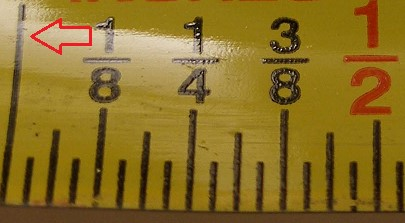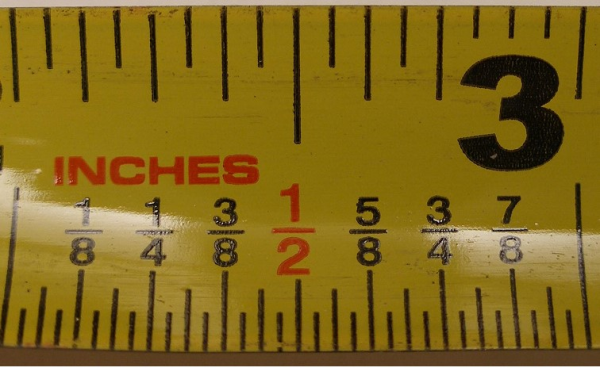Straight edges typically feature 1/16" increments.
1. Find the right length (size) of tool for the job. While a 72 inch straight edge could be used for measuring or drawing a 4 inch line, it makes more sense to use a shorter cutting guide tool. Using a 12 inch (standard foot ruler) will be easier and faster, as the foot-long is designed for lengths 12 inches and below.
2. Pay attention to the markings on the straight edge or ruler. The larger the mark, the bigger the increment and vica versa. On a standard 12 inch ruler, the largest markings indicate a full inch.
3. Smaller markings in between the inch marks indicate fractions of an inch, with the fraction size being directly proportional to the size of the mark. For example, the ½ inch mark (see below right) is shorter than the inch mark. The number of markings on a straight edge or ruler depends on how small an inch is broken down.
4. A ruler’s markings move left to right, as if you were reading a book. This means that on a standard foot ruler, one inch in the left edge and 12 inches is on the right edge. Keep that in mind when using a cutting guide tool.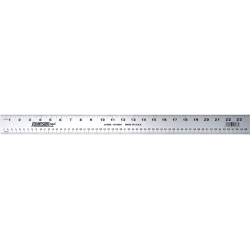## How is a Ruler Different from a Straight Edge

1. Look along the edges of the straight edge.
2. If there are markings indicating units of measurement along the edges, it is a ruler.
3. If there are no markings, it is simply a straight edge.
4. A ruler can be used for measuring and marking straight lines.
5. A straight edge will not help you measure, but most are built more durably than rulers, making them a superior tool for marking straight lines.
6. In most cases, rulers can function as a straight edge.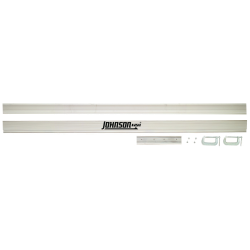## How to Use a Straight Edge

1. Use a straight edge to mark straight lines and as a guide for cutting.
2. Determine where you need to make your cut.
3. Mark one edge of the board at the desired length, and mark the other edge at the same length (or different, if you want an angled cut).
4. Line up each mark on one side of the straight edge.
5. Draw the pencil along the straight edge. Repeat as necessary to make sure the line is visible.
6. You have a perfectly straight line to cut along.
7. You may use the straight edge as a guide for your table saw (use c-clamps to secure the straight edge on the ends).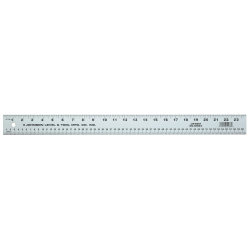## How to Use a Straight Edge with a Circular Saw

1. Assume you have made a straight line and are ready to make a cut.
2. Line up your circle saw blade along the line, but do not yet turn on the saw.
3. Make a mark where the saw base plate ends on the wood along the edge of the board.
4. Measure the distance from your cut line to where you marked the end of the base plate.
5. Go to the other side of the board. Use a construction square to make a mark at the same distance as close to the edge as possible.
6. Use your straight edge to mark the base plate line.
7. Line up the straight edge along the new base plate line.
8. If you are using an aluminum or metal straight edge, secure the straight edge in place using c-clamps on each end of the board.
9. If you are using a wooden guide as a straight edge (a 1x4 or 2x4), you can either use c-clamps or screw the guide directly into the board you're cutting—unless you plan on using the remainder of the board.
10. When the straight edge is secure, line up the blade with the cut line and ensure the straight edge is placed correctly.
11. Make the cut.